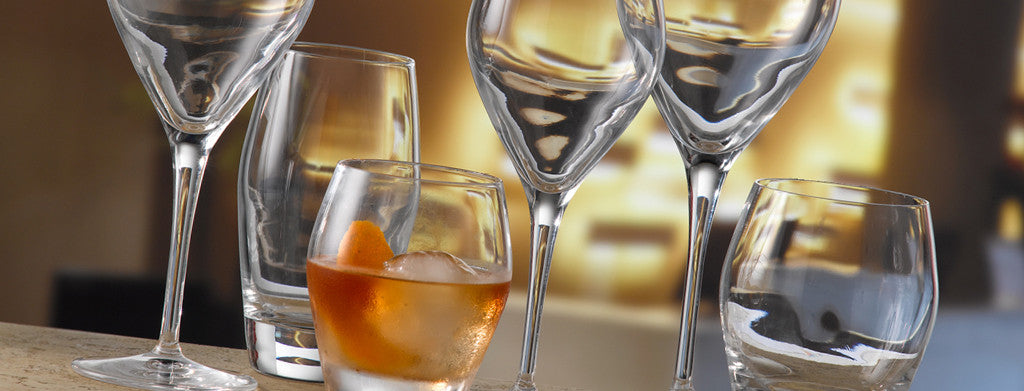Shopping Cart

### Refine

View all

#### Price# Glassware£1.39 each (ex VAT)£1.63 each (ex VAT)£1.53 each (ex VAT)£1.57 each (ex VAT)£1.40 each (ex VAT)£2.79 each (ex VAT)£1.64 each (ex VAT)£1.65 each (ex VAT)£1.30 each (ex VAT)£1.32 each (ex VAT)£1.46 each (ex VAT)£3.26 each (ex VAT)£2.82 each (ex VAT)£3.05 each (ex VAT)£1.64 each (ex VAT)£1.55 each (ex VAT)£1.32 each (ex VAT)£1.51 each (ex VAT)£1.44 each (ex VAT)£1.29 each (ex VAT)£1.44 each (ex VAT)£1.33 each (ex VAT)£1.36 each (ex VAT)£1.31 each (ex VAT)£1.53 each (ex VAT)£1.48 each (ex VAT)£18.45 each (ex VAT)£19.81 each (ex VAT)£19.66 each (ex VAT)£15.31 each (ex VAT)£1.58 each (ex VAT)£1.56 each (ex VAT)£3.11 each (ex VAT)£2.70 each (ex VAT)£3.14 each (ex VAT)£2.70 each (ex VAT)£3.14 each (ex VAT)£2.99 each (ex VAT)£1.58 each (ex VAT)